# One-Diode Model Temperature Correction

Given the 1-diode model parameters defined for the module under STC conditions (25 °C, 1000 W/m2), compute the temperature and irradiance-corrected parameters at the actual module temperature Tm and the available solar energy GT,Eff. The default temperature correction of all 1-diode parameters is linear. If a non-linear temperature correction of the diode ideality factor is desired, the then a set of additional parameters (polynomial coefficients) is available to affect this correction.

This non-linear response is illustrated in Figure 36 for a family of I(V) curves with a module temperature range of 8 °C to 75 °C. This data was measured in the laboratory using the module temperature control system and a Spire long-pulse solar simulator.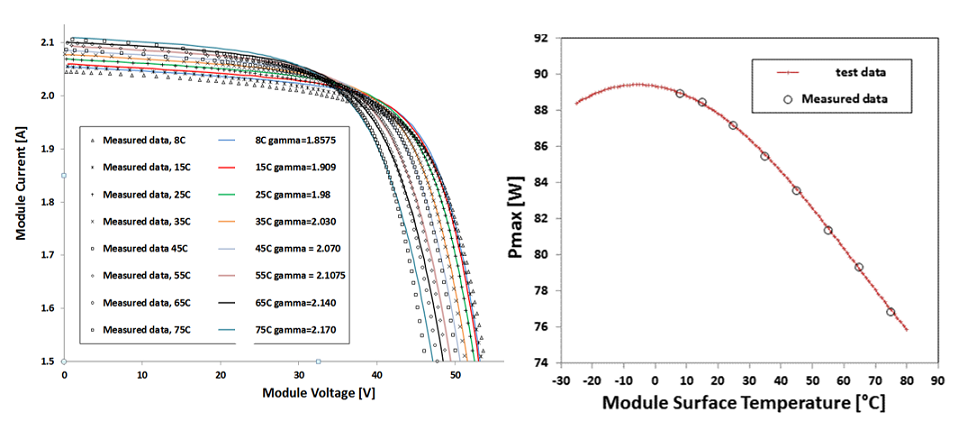## Inputs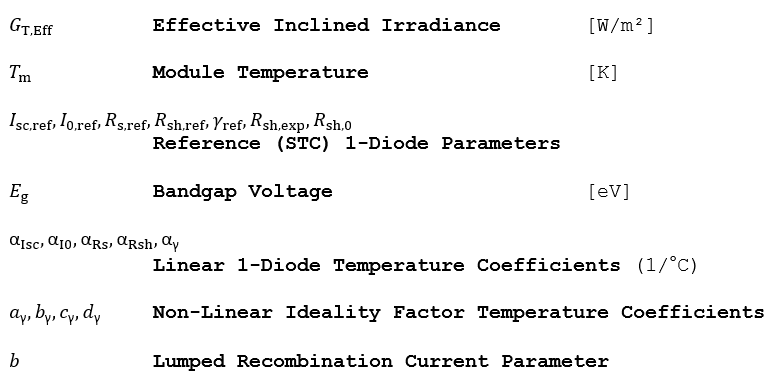## Outputs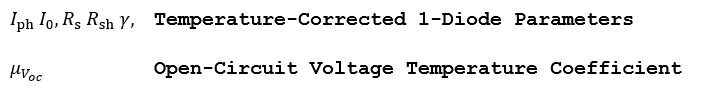## Algorithm

1.) Given the effective available insolation GT,Eff and module temperature Tm, find the corrected 1-diode shunt and series resistance.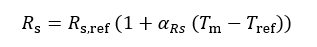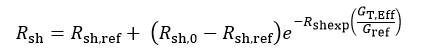2.) If the linear correction to the diode ideality factor is desired, or all non-linear coefficients ay,by,cy,dy = 0, then correct it for the module temperature as follows: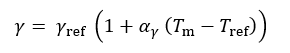3.) If the non-linear correction to the diode ideality factor is desired, then correct it for the module temperature as follows: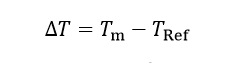4.) Correct the saturation current for temperature. Note that this correction includes the previously temperature-corrected diode ideality factor.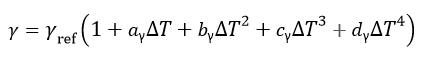*  In the above equation aγ is referring to the y1 non-linear temperature coefficient in PlantPredict and bγ is referring to y2, cγ is referring to y3 and dγ is referring to y45.) Find the short-circuit current under the ambient conditions.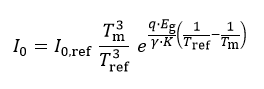6.) Even when a module is biased in short-circuit condition, a voltage drop occurs across Rs. As a result, the junction still experiences a voltage bias and there are currents flowing through both the recombination path and through Rsh when the terminal voltage of the device is zero, i.e. the recombination term does not go to zero in the short circuit condition. Therefore the photocurrent, Iph must be greater than Isc in order to supply parasitic currents as well as the external current which has the rated ISC,ref value. Find the actual Iph as follows: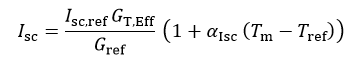The temperature dependence on voltage is also computed, but not used elsewhere in the simulation. Note that μΙsc ≅ αIph, given in 1/°C.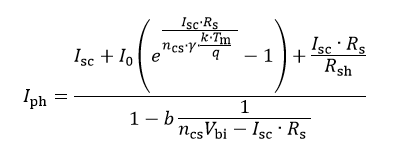## Reference

Schwieger, M., Michalksi, S., Non-Linearity of Temperature Coefficients, Equivalent Cell Temperature and Temperature Behaviour of Different PV-Module Technologies. TÜV Rheinland Energie und Umwelt, Cologne, Germany. Proceedings from the 28th EU PVSEC.